Courses

# Integer Value of The d- and f-Block Elements & Coordination Compounds, Past year Ques, JEE Adv. JEE Notes | EduRev

## Class 12 Chemistry 35 Years JEE Mains &Advance Past yr Paper

Created by: Abhishek Kapoor

## JEE : Integer Value of The d- and f-Block Elements & Coordination Compounds, Past year Ques, JEE Adv. JEE Notes | EduRev

The document Integer Value of The d- and f-Block Elements & Coordination Compounds, Past year Ques, JEE Adv. JEE Notes | EduRev is a part of the JEE Course Class 12 Chemistry 35 Years JEE Mains &Advance Past yr Paper.
All you need of JEE at this link: JEE

Q.1. The number of water molecule(s) directly bonded to the metal centre in CuSO4.5H2O is             (2009 - 4 Marks)

Ans. 4

Solution. The number of water molecules directly bonded to the metal centre in CuSO4.5H2O is 4.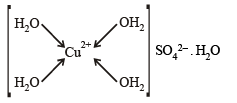Q.2. The oxidation number of Mn in the product of alkaline oxidative fusion of MnO2 is              (2009 - 4 Marks)

Ans. 6

Solution. 2MnO2 + 4KOH + O2 →2K 2MnO4+ 2H2O

Oxidation number of Mn in K2MnO4 is 6

K2MnO4 ;  2 + x – 8 = 0

x = 6

Q.3. Total number of geometrical isomers for the complex  [RhCl(CO) (PPh3) (NH3)] is            (2010)

Ans. 3

Solution.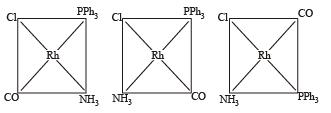The number of geometrical isomers is 3.

Q.4. The volume (in mL) of 0.1 M AgNO, required for complete precipitation of chloride ions present in 30 mL of 0.01 M solution of [Cr(H2O)5Cl]Cl2, as silver chloride is close to               (2011)

Ans. 6

Solution. m moles of [Cr (H2O)5Cl]Cl2 = 0.01 × 30 = 0.3
m moles of Cl–  = 0.3 × 2 = 0.6
[1 mole of complex gives 2 Cl ions]
m moles of Ag+ = m moles of Cl
0.1 × V = 0.6
V = 6 mL

Q.5. EDTA4– is eth ylen ediaminetetraacetate ion . Th e total number of N—Co—O bond angles in [Co(EDTA)]1– complex ion is              (JEE Adv. 2013)

Ans. 8

Solution.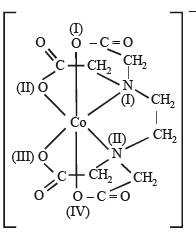Total no. of N – Co – O bonds are 8.

Q.6. Consider the following list of reagents: (JEE Adv. 2014)

Acidified K2Cr2O7, alkaline KMnO4, CuSO4, H2O2, Cl2, O3, FeCl3, HNO3 and Na2S2O3.

The total number of reagents that can oxidise aqueous iodide to iodine is

Ans. 7

Solution. K2Cr2O7 + KI + H2SO4 → K2SO4 + Cr2(SO4)3 + I2 + H2O

2CuSO4 + KI → 2CuI + I2 + 2K2SO4

H2O2 + 2KI → 2KOH + I2

Cl2 + 2KI → 2KCl + I2

O3 + H2O + 2KI → 2KOH + O2 + I2
FeCl3 + 2KI → 2KCl + FeCl2 + I2
HNO3 + KI → KNO3 + I2 + NO

Q.7. In the complex acetylbromidodicarbonylb is (triethylphosphine) iron (II), the number of Fe–C bond(s) is            (JEE Adv. 2015)

Ans. 3

Solution.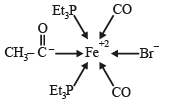Q.8. Among the complex ions, [Co(NH2–CH2–CH2–NH2)2Cl2]+, [CrCl2(C2O4)2]3–, [Fe(H2O)4(OH)2]+, [Fe(NH3)2(CN)4], [Co(NH2–CH2–CH2–NH2)2(NH3)Cl]2+ and [Co(NH3)4(H2O)Cl]2+, the number of complex ion(s) that show(s) cis-trans isomerism is                  (JEE Adv. 2015)

Ans. 6

Solution. All the complexes given show cis-trans isomerism

[Co (NH2 — CH2 — CH2 — NH2)2Cl2]+ [CrCl2(C2O4)2]3–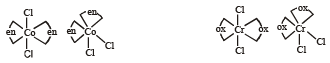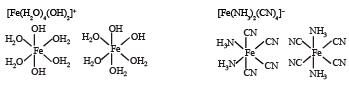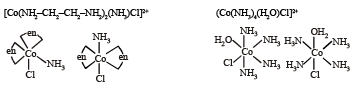Q.9. For the octahedral complexes of Fe3+ in SCN (thiocyanatoS) and in CN ligand environments, the difference between the spin-only magnetic moments in Bohr magnetons (when approximated to the nearest integer) is

[Atomic number of Fe = 26]                      (JEE Adv. 2015)

Ans. 4

Solution.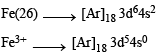SCN is weak field ligand hence pairing will not occur.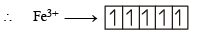Unpaired electrons = 5

Magnetic moment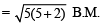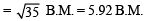CN is strong field ligand hence pairing will take place.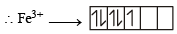Unpaired electrons = 1

Magnetic moment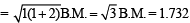Difference = 5.92 – 1.732 = 4.188

Q.10. In neutral or faintly alkaline solution, 8 moles of permanganate anion quantitatively oxidize thiosulphate anions to produce X moles of a sulphur containing product. The magnitude of X is                     (JEE Adv. 2016)

Ans. 6

Solution.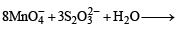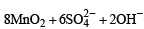∴ 8 moles of MnO4 form 6 moles of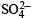Q.11. The number of geometric isomers possible for the complex [CoL2Cl2] (L = H2NCH2CH2O) is                    (JEE Adv. 2016)

Ans. 5

Solution. [CoL2Cl2]   (L = H2NCH2CH2O)

L is unsymmetrical didentate ligand.

So the complete is equivalent to [M(AB)2a2] Possible G..I. are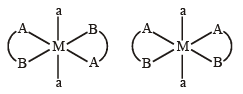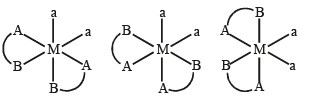Offer running on EduRev: Apply code STAYHOME200 to get INR 200 off on our premium plan EduRev Infinity!

69 docs|36 tests

,

,

,

,

,

,

,

,

,

,

,

,

,

,

,

,

,

,

,

,

,

,

,

,

,

,

,

;